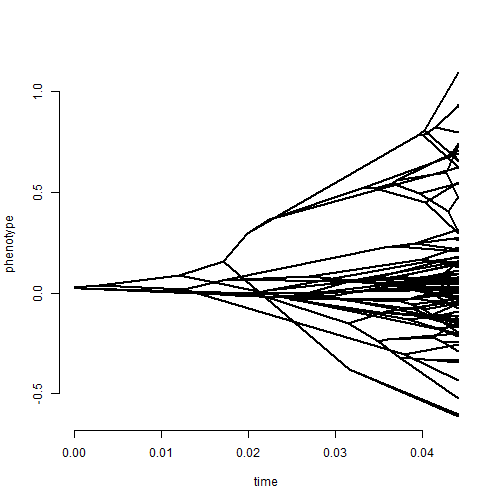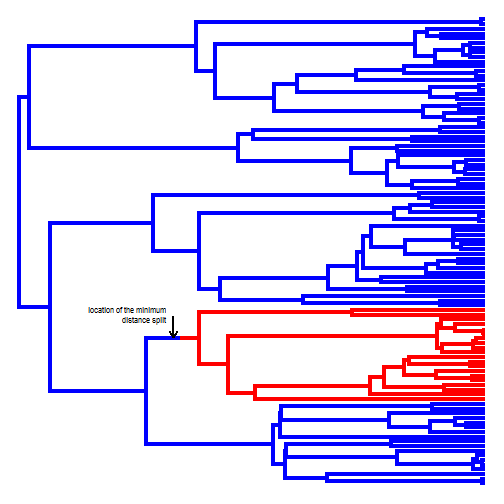## Wednesday, November 8, 2017

### (New) `print` method for (old) Bayesian MCMC function to identify the phylogenetic position of a rate-shift

A number of years ago some colleagues & I described a new Bayesian method (Revell et al. 2012) for identifying shifts in the rate of phenotypic evolution on a phylogeny.

Believe it or not, at the time we thought that this was cutting-edge - and in a sense it was. To that point, there had been very limited use of Bayesian MCMC in phylogenetic comparative methods, particularly for continuous traits. Furthermore, though techniques to test a priori hypotheses about changes in the evolutionary rate or process for continuous traits were already well-developed, no one (at that point) had published a technique for identifying shifts absent a prior hypothesis. This, of course, changed rapidly as members of the Harmon Lab at the University of Idaho published various rjMCMC (reversible-jump) Bayesian methods designed to sample rates and the location of rate-shifts from their joint posterior distribution.

Nonetheless, our method is still valid. The function implementing the method, `evol.rate.mcmc`, has long been part of the phytools package. Today, I decided to create a custom object class & add a `print` method to the function - just to make it a little easier to use.

Here's a quick demo:

``````library(phytools)
``````
``````## Loading required package: ape
``````
``````## Loading required package: maps
``````
``````phenogram(tree,x,ftype="off")
````````````mcmc<-evol.rate.mcmc(tree,x,ngen=100000,quiet=TRUE)
``````
``````## Starting MCMC run....
``````
``````## Done MCMC run.
``````
``````mcmc
``````
``````##
## Object of class "evol.rate.mcmc" containing the results from a
## the Bayesian MCMC analysis of a Brownian-motion rate-shift model.
##
## MCMC was conducted for 1e+05 generations sampling every 100
## generations.
##
## The most commonly sampled rate shift(s) occurred on the edge(s)
## to node(s) 121.
##
## Use the functions posterior.evolrate and minSplit for more detailed
## analysis of the posterior sample from this analysis.
``````

In fact, the method has easily identified the precise edge on which I simulated a rate-shift. Here is a plot showing the simulated shift & then I will calculate the rate-shift location with the minimum distance to the rest in the posterior sample & overlay it.

``````plot(mtree,colors=setNames(c("blue","red"),1:2),ftype="off",lwd=4)
split<-minSplit(tree,mcmc\$mcmc[201:nrow(mcmc\$mcmc),c("node","bp")])
lastPP<-get("last_plot.phylo",envir=.PlotPhyloEnv)
xx<-rep(nodeheight(tree,getParent(tree,split\$node)),2)+split\$bp
yy<-lastPP\$yy[split\$node]+c(0,0.05*Ntip(tree))
library(plotrix)
p2p_arrows(xx,yy,xx,yy,lwd=2,length=0.1,col="grey")
text(xx,yy,"location of the minimum\ndistance split",pos=2,
cex=0.7)
``````Neat - we're almost right on.

I simulated the tree & data as follows (although I ran it a few times to ensure that the shift was not on a terminal edge or for a very small clade):

``````foo<-function(){
obj<-pbtree(n=100)
obj\$edge.length<-obj\$edge.length/sum(obj\$edge.length)
obj
}
Q<-matrix(c(-1,1,1,-1),2,2)
nchanges<-function(tree) sum(sapply(tree\$maps,length))-nrow(tree\$edge)
mtree<-sim.history(tree<-foo(),Q)
while(nchanges(mtree)!=1) nchanges(mtree<-sim.history(tree<-foo(),Q))
x<-sim.rates(mtree,setNames(c(1,10),1:2))
``````